# Complex number

(Redirected from Real part)

Complex numbers are numbers of the form$a+b\cdot i$, where a and b are real numbers and i denotes a number satisfying$i^{2}=-1$. Of course, since the square of any real number is nonnegative, i cannot be a real number. At first glance, it is not even clear whether such an object exists and can be reasonably called a number; for example, can we sensibly associate with i natural operations such as addition and multiplication? As it happens, we can define mathematical operations for these "complex numbers" in a consistent and sensible way and, perhaps more importantly, using complex numbers provides mathematicians, physicists, and engineers with an extremely powerful approach to expressing parts of these sciences in a convenient and natural way.

##  Historical development

A common complaint among math students is why they must bother with complex numbers when real numbers almost always seem sufficient for applications. Indeed, many familiar real world quantities, such as distance, temperature, and time are best described using real numbers. In contrast, although there are many physical situations which are mostly aptly described with complex numbers, most of these situations require some relatively sophisticated knowledge of physics, and the phenomena which they describe are more abstruse than distance, temperature, or time.

Many modern students first encounter complex numbers when solving quadratic equations, which can have complex number solutions. This presentation is historically misleading — the quadratic formula precedes recognition of the utility of complex numbers by many centuries. The ancients could simply dismiss quadratic equations whose solutions involve complex numbers as having no real roots.

The need for complex numbers might have appeared for the first time during the sixteenth century, when Italian mathematicians like Scipione del Ferro, Niccolò Fontana Tartaglia, Gerolamo Cardano and Rafael Bombelli tried to solve cubic equations. Even for equations with three real solutions, the method they used sometimes required calculations with numbers whose squares are negative. A historical example of this can be found on the "advanced" subpage for this article.

In modernity, complex numbers form the basis for the mathematical models of many physical phenomena, including electro-magnetism and quantum mechanics. Of course, an equivalent mathematical model can be formulated using pairs of real numbers instead, which however is less heuristic. (Formally, all mathematical theories boil down to sets, but no human could understand and use these theories after such reduction.)

## Working with complex numbers

As a first step in giving some legitimacy to the "number"$\sqrt{-1}$, we will explain how to compute with it. How do you add, multiply and divide expressions with this number? It turns out that this is not that difficult; the main rule to keep in mind is that the square of$\sqrt{-1}$ equals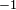$-1$.

In the remainder of the article, we will use the letter i to denote one solution of the equation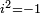$i^2 = -1$, where we previously used$\sqrt{-1}$. With this convention, all complex numbers can be written as a + bi, where a and b are real numbers. We call a the real part of the complex number and b the imaginary part. The complex number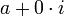$a + 0\cdot i$ whose imaginary part is zero is considered to be the same thing as the real number a.

### Basic operations

Addition of complex numbers is straightforward,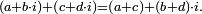$(a + b\cdot i) + (c + d\cdot i) = (a + c) + (b + d) \cdot i.$ The result is again a complex number.

Multiplication is more interesting. Suppose we want to compute$(a+b \cdot i)\cdot(c+d\cdot i)$. Using$i^2 = -1$, we can rewrite this product in a form which clearly shows it to be another complex number:$(a + b\cdot i)\cdot (c + d\cdot i) = a\cdot c + a\cdot d\cdot i + b\cdot c\cdot i + b\cdot d\cdot i^2 = (a\cdot c - b\cdot d) + (b\cdot c + a\cdot d)i. \$

To handle division, we simply note that$(c + d\cdot i)\cdot (c - d\cdot i) = c^2 +d^2$, so, provided that c and d are not simultaneously zero,$\frac{1}{c + d\cdot i} = \frac{c - d\cdot i}{c^2 + d^2},$

from which it follows that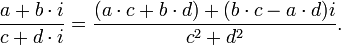$\frac{a + b\cdot i}{c + d\cdot i} = \frac{(a\cdot c + b\cdot d) + (b\cdot c - a\cdot d)i}{c^2 + d^2}.$

If c = d = 0 then division by$c+d\cdot i$ is not defined.

Going a bit further, we can introduce the important operation of complex conjugation. Given an arbitrary complex number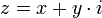$z = x + y\cdot i$, we define its complex conjugate to be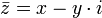$\bar{z} = x - y \cdot i$. Using the identity$(a + b)\cdot(a - b) = a^2 - b^2$ we derive the important formula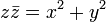$z \bar{z} = x^2 + y^2$

and we define the modulus of a complex number z to be$|z| = \sqrt{z \bar{z}}.$

Note that the modulus of a complex number is always a nonnegative real number. The modulus (also called absolute value) satisfies three important properties that are completely analogous to the properties of the absolute value of real numbers:

•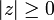$|z| \ge 0$; furthermore, | z | = 0 if and only if z = 0
•$|z_1 z_2| = |z_1| |z_2| \$
•$|z_1 + z_2 | \le |z_1| + |z_2|$

The last inequality is known as the triangle inequality.

### The complex exponential

Recall that in real analysis, the ordinary exponential function may be defined as$e^x = 1 + x + \frac{x^2}{2!} + \frac{x^3}{3!} + \cdots$

The same series may be used to define the complex exponential function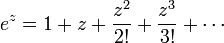$e^z = 1 + z + \frac{z^2}{2!} + \frac{z^3}{3!} + \cdots$

(where, of course, convergence is defined in terms of the complex modulus, instead of the real absolute value).

The complex exponential has the same multiplicative property that holds for real numbers, namely$e^{z_1 + z_2} = e^{z_1} e^{z_2} \$

The complex exponential function has the important property that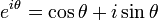$e^{i\theta} = \cos \theta + i \sin \theta \$

as may be seen immediately by substituting z = iθ and comparing terms with the usual power series expansions of sinθ and cosθ.

The familiar trigonometric identity$\sin^2 \theta + \cos^2 \theta = 1 \$

immediately implies the important formula

| eiθ | = 1, for any$\theta \in \mathbb{R}.$

Another way to establish this identity is to note that$\overline{e^{i\theta}} = e^{-i\theta}$, so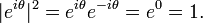$|e^{i\theta}|^2 = e^{i\theta}e^{-i\theta} = e^0 = 1. \$

### Geometric interpretation

Since a complex number z = x + iy is specified by two real numbers, namely x and y, it can be interpreted as the point (x,y) in the plane. When complex numbers are represented as points in the plane, the resulting diagrams are known as Argand diagrams, after Robert Argand. The geometric representation of complex numbers turns out to be very useful, both as an aid to understanding the properties of complex numbers and as a tool in applying complex numbers to geometrical and physical problems.

There are no real surprises when we look at addition and subtraction in isolation: addition of complex numbers is not essentially different from addition of vectors in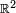$\mathbb{R}^2$. Similarly, if$\alpha \in \mathbb{R}$ is real, multiplication by α is just scalar multiplication. In$\mathbb{C}$ we have$z_1 + z_2 = (x_1 + iy_1) + (x_2 + iy_2) = (x_1 + x_2) + i(y_1 + y_2) \$

and$\alpha z = \alpha(x + i \cdot y) = \alpha x + i\alpha y. \,$

To put it succinctly,$\mathbb{C}$ is a 2-dimensional real vector space with respect to the usual operations of addition of complex numbers and multiplication by a real number. There doesn't seem to be much more to say. But there is more to say, and that is that the multiplication of complex numbers has geometric significance. This is most easily seen if we take advantage of the complex exponential, and write complex numbers in polar form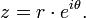$z = r\cdot e^{i\theta}.$

Here, r is simply the modulus$|z| = \sqrt{x^2 + y^2}$ or vector length. The number θ is just the angle formed with the x-axis, and is called the argument. Now, when complex numbers are written in polar form, multiplication is very interesting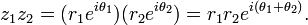$z_1 z_2 = (r_1 e^{i\theta_1}) (r_2 e^{i\theta_2}) = r_1 r_2 e^{i(\theta_1 + \theta_2)}.$

In other words, multiplication by a complex number z has the effect of simultaneously scaling by the number's modulus and rotating by its argument. This is really astounding. For example, to multiply a given complex number z by i we need only to rotate z by π / 2 (that is, 90 degrees). Translation corresponds to complex addition, scaling to multiplication by a real number, and rotation to multiplication by a complex number of unit modulus. The one type of coordinate transformation that is missing from this list is reflection. On the other hand, there is an arithmetic operation we have not considered, and that is division. Recall that for non-zero z$\frac{1}{z} = \frac{\bar{z}}{|z|^2}.$

Division of a complex number z1 by a non-zero complex number z2 can then be interpreted as multiplication of z1 by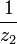$\frac{1}{z_2}$. This in turn corresponds to scaling of the modulus of z1 by the inverse of the modulus of z2 and a rotation of its argument by the negative of the argument of z2. That is,$\frac{z_1}{z_2}=z_1 (\frac{1}{z_2})=\frac{1}{|z_2|^2}z_1 \overline{z_2}=\frac{|z_1|}{|z_2|}e^{i(\theta_1-\theta_2)},$

where θ12 are the arguments of z1,z2, respectively.

Returning to the representation of complex numbers in rectangular form, we note that complex conjugation is just the transformation (or map)$x + iy \;\mapsto\; x - iy$ or, in vector notation,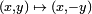$(x, y)\; \mapsto \;(x, -y)$. This is nothing other than reflection in the x-axis, and any other reflection may be obtained by combining that transformation with rotations and translations.

Historically, this observation was very important and led to the search for higher dimensional algebras that could "arithmetize" Euclidean geometry. It turns out that there are such generalizations in dimensions 4 and 8, known as the quaternions and octonions (also known as Cayley numbers). At that point, the process stops, but the ideas developed in this process have played an important role in the development of modern differential geometry and mathematical physics.

## Algebraic closure

An important property of the set of complex numbers is that it is algebraically closed. This means that any non-constant polynomial with complex coefficients has a complex root. This result is known as the Fundamental Theorem of Algebra.

This is actually quite remarkable. We started out with the real numbers. There are many polynomials with real coefficients that do not have a real root. We took just one of these, the polynomial x2 + 1, and we introduced a new number, i, which is defined to be a root of the polynomial. Suddenly, all non-constant polynomials have a root in this new setting where we allow complex numbers.

There are many proofs of the Fundamental Theorem of Algebra. Many of the simplest depend crucially on complex analysis. But it is by no means necessary to rely on complex analysis here. A proof using field theory is alluded to at the very end of this article.

## Formal definition

We have been treating complex numbers very much like real numbers and found that they can be very useful, but we have not yet proven that they exist or that they can be used without running into contradictions. In fact, it is quite easy to go wrong when using complex numbers. Consider for instance the following computation:$-1=\sqrt{-1}\times\sqrt{-1}=\sqrt{(-1)\times(-1)}=\sqrt{1}=1.$

This computation seems to show that − 1 equals 1, which is nonsense. The point is that the second equality can not be applied. Positive real numbers satisfy the identity$\sqrt{a}\times\sqrt{b} = \sqrt{a \times b},$

but this identity does not hold for negative real numbers, whose square roots are not real, because the square root symbol denotes only the positive solution to x2 = a.

One possibility to feel more secure when using complex numbers is to define them in terms of constructs which are better understood. This approach was taken by Hamilton, who defined complex numbers as ordered pairs of real numbers, that is,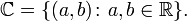$\mathbb{C}= \{ (a,b) \colon a,b\in \mathbb{R} \}.$

Addition and multiplication of such pairs can be defined as follows:

• addition: (a,b) + (c,d) = (a + c,b + d)
• multiplication: (a,b)(c,d) = (acbd,bc + ad)

The multiplication may look artificial, but it is inspired by the formula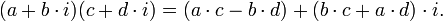$(a + b\cdot i)(c + d\cdot i) = (a\cdot c - b\cdot d) + (b\cdot c + a\cdot d)\cdot i. \$

which we derived before.

These definitions satisfy most of the basic properties of addition and multiplication of real numbers, and we can employ many formulas from the elementary algebra we are accustomed to. More specifically, it can easily be shown that addition and multiplication as defined above are commutative and associative, and that multiplication is distributive over addition; in other words, the sum (or the product) of two numbers does not depend on the order of terms; the sum (product) of three or more elements does not depend on order of operations ('we can suppress the parentheses'); the product of a complex number with a sum of two other numbers expands in the usual way. In mathematical language this means that with addition and multiplication defined this way,$\mathbb{C}$ satisfies the axioms for a ring, and moreover a field, and is called the field of complex numbers.

Now we are ready to understand the 'real' meaning of i. Observe that the pairs of type (a,0) are identical to the set of reals, so we write (a,0) = a. Observe also that by definition (0,1)(0,1) = ( − 1,0) = − 1. In other words, we can define i, the symbol we've been using, as the pair (0,1). In this way we have a way of indicating which one we mean of the two solutions of the equation i2 = − 1; the other is now denoted (0,-1).

Another way to define the complex numbers comes from field theory. Because x2 + 1 is irreducible in the polynomial ring$\mathbb{R}[x]$, the ideal generated by x2 + 1 is a maximal ideal. Therefore, the quotient ring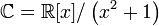$\mathbb{C}=\mathbb{R}[x]/\left(x^2+1\right)$ is a field. We can choose the polynomials of degree at most 1 as the representatives for the equivalence classes in this quotient ring. So in a sense, we can imagine that the dummy variable x is the imaginary number i, and the elements of the quotient ring behave exactly the way we expect the complex numbers to behave. For example, x2 is in the same equivalence class as − 1, and so x2 = − 1 in this quotient ring. (As a final comment in this analysis, we could next show that$\mathbb{C}$ has no finite extension and must therefore be algebraically closed.)

## Notes and references

1. This article follows the usual convention in mathematics and physics of using i as the imaginary unit. Complex numbers are frequently used in electrical engineering, but in that discipline it is usual to use j instead, reserving i for electrical current. This usage is found in some programming languages too, notably Python.
2. Part of the reason for not using$\sqrt{-1}$ is that the symbol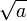$\sqrt{a}$ (or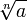$\sqrt[n]{a}$) with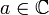$a\in\mathbb{C}$ is sometimes used to denote the set of complex roots of a, i.e., the set of the solutions of the equation x2 = a (xn = a respectively). The set contains 2 (n, respectively) "equally important" elements and there is no canonical way to distinguish a "representative". Consequently, no computations are performed using this symbol.
3. that is, the addition (multiplication) is commutative
4. This is called associativity
5. In other words, multiplication is distributive over addition
6. i.e., isomorphic, which basically means that the mapping$\mathbb{C}\ni (a,0)\mapsto a\in\mathbb{R},$ preserves the addition and multiplication.
7. An ideal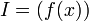$I = \left(f(x)\right)$ in a polynomial ring over a field is maximal if and only if f(x) is irreducible over the field.Some content on this page may previously have appeared on Citizendium; see the talk page.